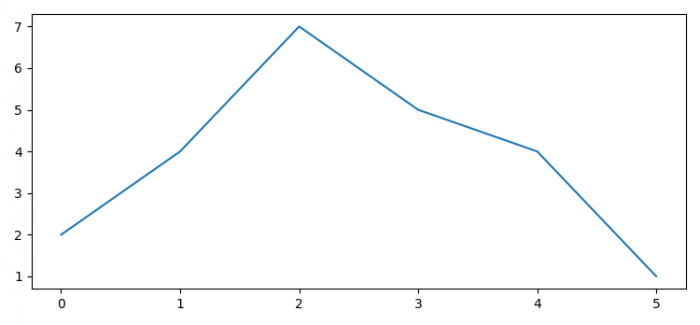# How do I show the same Matplotlib figure several times in a single IPython notebook?

To show the same Matplotlib figure several times in a single iPython notebook, we can take the following steps −

• Set the figure size and adjust the padding between and around the subplots.
• Create a figure and a set of subplots.
• Plot the data points on that axes.
• To show the current figure again, use fig.show() method.

## Example

In : %matplotlib auto
Using matplotlib backend: Qt5Agg

In : import matplotlib.pyplot as plt

In : plt.rcParams["figure.figsize"] = [7.50, 3.50]
...: plt.rcParams["figure.autolayout"] = True

In : fig, ax = plt.subplots()

In : ax.plot([2, 4, 7, 5, 4, 1])
Out: [<matplotlib.lines.Line2D at 0x7f4270361c50>]

In : fig.show()

## Output